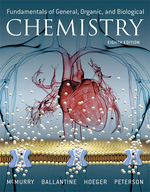×
Get Full Access to Fundamentals Of General, Organic, And Biological Chemistry (Mastering Chemistry) - 8 Edition - Chapter 5 - Problem 5.65
Get Full Access to Fundamentals Of General, Organic, And Biological Chemistry (Mastering Chemistry) - 8 Edition - Chapter 5 - Problem 5.65

×ISBN: 9780134015187 2044

## Solution for problem 5.65 Chapter 5

Fundamentals of General, Organic, and Biological Chemistry (Mastering Chemistry) | 8th Edition

• Textbook Solutions
• 2901 Step-by-step solutions solved by professors and subject experts
• Get 24/7 help from StudySoup virtual teaching assistantsFundamentals of General, Organic, and Biological Chemistry (Mastering Chemistry) | 8th Edition

4 5 1 254 Reviews
20
0
Problem 5.65

In the Breathalyzer test, blood alcohol is determined by reaction of the alcohol with potassium dichromate:

\begin{aligned} 16 \mathrm{H}^{+}(a q)+2 \mathrm{Cr}_{2} \mathrm{O}_{7}^{2-}(a q)+\mathrm{C}_{2} \mathrm{H}_{5} \mathrm{OH}(a q) & \longrightarrow \\ 4 \mathrm{Cr}^{3+}(a q)+2 \mathrm{CO}_{2}(g)+11 \mathrm{H}_{2} \mathrm{O}(l) \end{aligned}

(a) Calculate the oxidation number of Cr in$$\mathrm{Cr}_{2} \mathrm{O}_{7}^{2-}$$.

(b) Calculate the oxidation number of C in $$\mathrm{C}_{2} \mathrm{H}_{5} \mathrm{OH}$$ and in $$\mathrm{CO}_{2}$$.

(c) Identify the oxidizing agent and the reducing agent in this reaction.

Text Transcription:

16H+ (aq) + 2Cr_2O_7^2- (aq) + C_2H_5OH (Aq) arrow right 4 Cr^3+ (aq) + 2 Co_2 (g) 11 H_2O (i)

Cr_2O_7^2-

C_2H_5OH

CO_2

Step-by-Step Solution:
Step 1 of 3

Chapter 6 - Power function y=bx^m gives a straight line when plotted on a log-log axis - Exponential function y=be^(mx) also y=b(10)^(mx) gives a straight line when plotted on a semilog plot where the y axis is logarithmic. - Exponential functions never pass through the origin - Power function can pass through origin only if M>0 - P=polyfit(x,y,n) returns n+1 terms - Least square criterion: minimizes the sum of the squares of the residuals (sort of like a line of best fit) - Polyfit is based on the least square method

Step 2 of 3

Step 3 of 3

##### ISBN: 9780134015187

This full solution covers the following key subjects: . This expansive textbook survival guide covers 281 chapters, and 456 solutions. The answer to “?In the Breathalyzer test, blood alcohol is determined by reaction of the alcohol with potassium dichromate: \begin{aligned}16 \mathrm{H}^{+}(a q)+2 \mathrm{Cr}_{2} \mathrm{O}_{7}^{2-}(a q)+\mathrm{C}_{2} \mathrm{H}_{5} \mathrm{OH}(a q) & \longrightarrow \\4 \mathrm{Cr}^{3+}(a q)+2 \mathrm{CO}_{2}(g)+11 \mathrm{H}_{2} \mathrm{O}(l)\end{aligned}(a) Calculate the oxidation number of Cr in$$\mathrm{Cr}_{2} \mathrm{O}_{7}^{2-}$$. (b) Calculate the oxidation number of C in $$\mathrm{C}_{2} \mathrm{H}_{5} \mathrm{OH}$$ and in $$\mathrm{CO}_{2}$$. (c) Identify the oxidizing agent and the reducing agent in this reaction.Text Transcription:16H+ (aq) + 2Cr_2O_7^2- (aq) + C_2H_5OH (Aq) arrow right 4 Cr^3+ (aq) + 2 Co_2 (g) 11 H_2O (i)Cr_2O_7^2-C_2H_5OHCO_2” is broken down into a number of easy to follow steps, and 87 words. This textbook survival guide was created for the textbook: Fundamentals of General, Organic, and Biological Chemistry (Mastering Chemistry), edition: 8. Since the solution to 5.65 from 5 chapter was answered, more than 200 students have viewed the full step-by-step answer. Fundamentals of General, Organic, and Biological Chemistry (Mastering Chemistry) was written by Aimee Notetaker and is associated to the ISBN: 9780134015187. The full step-by-step solution to problem: 5.65 from chapter: 5 was answered by Aimee Notetaker, our top Chemistry solution expert on 04/25/22, 03:57PM.

## Discover and learn what students are asking

Calculus: Early Transcendental Functions : Space Coordinates and Vectors in Space
?In Exercises 1-4, plot the points in the same three-dimensional coordinate system. (a) (2, 1, 3) (b) (-1, 2, 1)

Statistics: Informed Decisions Using Data : Tests for Independence and the Homogeneity of Proportions
?The following table contains the number of successes and failures for three categories of a variable. Test whether the proportions are

Statistics: Informed Decisions Using Data : Comparing Three or More Means (One-Way Analysis of Variance)
?Corn Production The data in the table represent the number of corn plants in randomly sampled rows (a 17-foot by 5-inch strip) for various types of pl

Unlock Textbook Solution